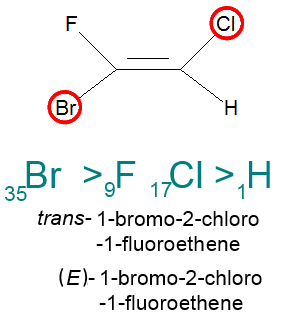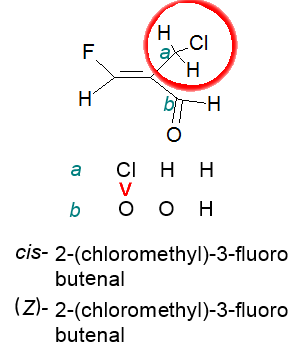# Nomenclature of cis-trans isomers

## Definition

For the most important groups or atoms attached to the two C=C carbon atoms: (Z) = cis = on the same side of the axis C=C (E) = trans = across the axis C=C

Example: Maleic acid = (Z)-butenedioic acid Fumaric acid = (E)-butenedioic acid

But what are the most important groups?

## Order of precedence

1) Comparing the two atoms directly attached to each carbon: The most important is the one with the highest atomic number!2) If the two atoms with the highest atomic number are the same, we classify atoms bound in turn to these two atoms in order of decreasing importance and compare from left to right. For a double bond, we take twice the atom bound.